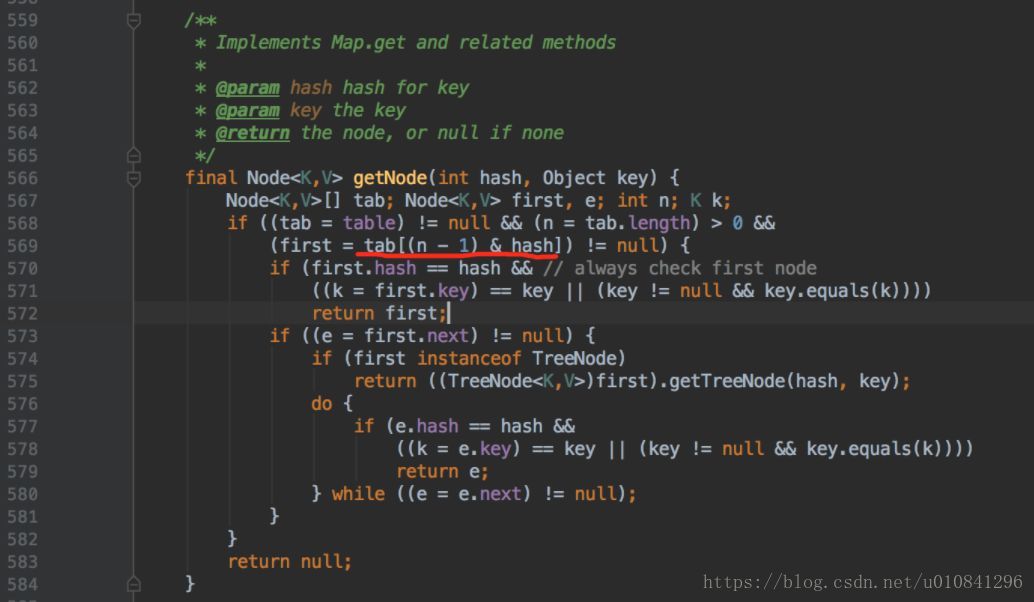HashMap是根据key的hash值决策key放入到哪个桶（bucket）中，通过 tab=[(n – 1) & hash] 公式计算得出，其中tab是一个哈希表。

1. 为什么要保证 capacity 是2的次幂呢？

1）在get方法实现中，实际上是匹配链表中的 Node[] tab 中的数据。

(n – 1) & hash实际上是计算出 key 在 tab 中索引位置，当key的hash没有冲突时，key在HashMap存储的位置就是匹配的node中的第一个节点。如果hash有冲突，就会在node里面节点中查询，直至匹配到相等的key。2）因为 n 永远是2的次幂，所以 n-1 通过 二进制表示，永远都是尾端以连续1的形式表示（00001111，00000011）

• &运算速度快，至少比%取模运算块

• 能保证 索引值 肯定在 capacity 中，不会超出数组长度

• (n – 1) & hash，当n为2次幂时，会满足一个公式：(n – 1) & hash = hash % n

2.为什么要通过 (n – 1) & hash 决定桶的索引呢？

1）key具体应该在哪个桶中，肯定要和key挂钩的，HashMap顾名思义就是通过hash算法高效的把存储的数据查询出来，所以HashMap的所有get 和 set 的操作都和hash相关。

2）既然是通过hash的方式，那么不可避免的会出现hash冲突的场景。hash冲突就是指 2个key 通过hash算法得出的哈希值是相等的。hash冲突是不可避免的，所以如何尽量避免hash冲突，或者在hash冲突时如何高效定位到数据的真实存储位置就是HashMap中最核心的部分。

3）首先要提的一点是 HashMap中 capacity 可以在构造函数中指定，如果不指定默认是2 的 (n = 4) 次方，即16。

public HashMap(int initialCapacity) {
}


4）HashMap中的hash也做了比较特别的处理，(h = key.hashCode()) ^ (h >>> 16)。

00000000 00001111 01000010 01000000

00000000 00000000 00000000 00001111

00000000 00001111 01000010 01001111

static final int hash(Object key) {
int h;
return (key == null) ? 0 : (h = key.hashCode()) ^ (h >>> 16);
}


3.capacity 永远都是 2 次幂，那么如果我们指定 initialCapacity 不为 2次幂时呢，是不是就破坏了这个规则？

/**
* Returns a power of two size for the given target capacity.
*/
static final int tableSizeFor(int cap) {
//cap-1后，n的二进制最右一位肯定和cap的最右一位不同，即一个为0，一个为1，例如cap=17（00010001），n=cap-1=16（00010000）
int n = cap - 1;
//n = (00010000 | 00001000) = 00011000
n |= n >>> 1;
//n = (00011000 | 00000110) = 00011110
n |= n >>> 2;
//n = (00011110 | 00000001) = 00011111
n |= n >>> 4;
//n = (00011111 | 00000000) = 00011111
n |= n >>> 8;
//n = (00011111 | 00000000) = 00011111
n |= n >>> 16;
//n = 00011111 = 31
//n = 31 + 1 = 32, 即最终的cap = 32 = 2 的 (n=5)次方
return (n < 0) ? 1 : (n >= MAXIMUM_CAPACITY) ? MAXIMUM_CAPACITY : n + 1;
}